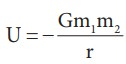Home | | Physics 11th std | Summary - Physics: Gravitation

# Summary - Physics: Gravitation

The motion of planets can be explained using KeplerŌĆÖs laws.

SUMMARY

The motion of planets can be explained using KeplerŌĆÖs laws.

KeplerŌĆÖs first law: All the planets in the solar system orbit the Sun in elliptical orbits with the Sun at one of the foci.

KeplerŌĆÖs second law: The radial vector line joining the Sun to a planet sweeps equal areas in equal intervals of time.

KeplerŌĆÖs third law: The ratio of the square of the time period of planet to the cubic power of semi major axis is constant for all the planets in the solar system.

NewtonŌĆÖs law of gravitation states that the gravitational force between two masses is directly proportional to product of masses and inversely proportional to square of the distance between the masses. In vector form it is given byGravitational force is a central force.

KeplerŌĆÖs laws can be derived from NewtonŌĆÖs law of gravitation.

The gravitational field due to a mass m at a point which is at a distance r from mass m is given by. It is a vector quantity.

The gravitational potential energy of two masses is given byIt is a scalar quantity.

The gravitational potential at a point which is at a distance r from mass m is given by. It is a scalar quantity.

Due to rotation of the Earth, the acceleration due to gravity is maximum at poles and minimum at EarthŌĆÖs equator.

The (escape) speed of any object required to escape from the EarthŌĆÖs gravitational field is ve = ŌłÜ2gRe . It is independent of mass of the object.

The energy of the satellite is negative. It implies that the satellite is bound to EarthŌĆÖs gravitational force.

Copernicus model explained that retrograde motion is due to relative motion between planets. This explanation is simpler than PtolemyŌĆÖs epicycle explanation which is complicated

Copernicus and Kepler measured the distance between a planet and the Sun using simple geometry and trigonometry.

2400 years ago, Eratosthenes measured the radius of the Earth using simple geometry and trigonometry.

Study Material, Lecturing Notes, Assignment, Reference, Wiki description explanation, brief detail
11th Physics : UNIT 6 : Gravitation : Summary - Physics: Gravitation |